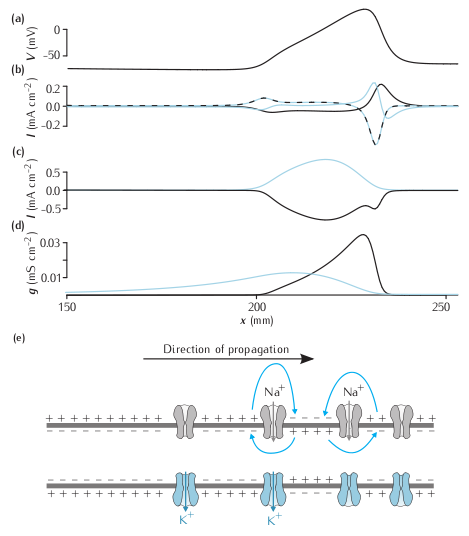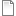# Figure 3.15Propagating action potential

Currents flowing during a propagating action potential. (a) the voltage along an axon at one instant in time during a propagating action potential. (b) The axial current (blue line), the ionic current (dashed black-blue line) and the capacitative current (black line) at the same points. (c) The sodium (black) and potassium (blue) contributions to the ionic current. The leak current is not shown. (d) The sodium (black) and potassium (blue) currents. (e) Representation of the state of ion channels, the membrane and local circuit current along the axon during a propagating action potential.

Simulation environment:
Notes

Due to the constraints of NEURON, not all the currents in the plot from the book are displayed by NEURON as the simulation proceeds. Of the currents in (b) in the figure, only  the capacitative current (i_cap in NEURON) is plotted directly by NEURON. Both the sodium and potassium currents from  (c) are shown directly (ina and ik). The total ionic current in  (b) is obtained by summing the sodium, potassium and leak currents (ina, ik and il_hh). The axial current is obtained using the equation I = -IC - Ii .fig-3-15.nrnzip